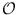Next: Basic Data Types for Up: Graphs and Iterators Previous: Strongly Connected Components (flexible)   Contents   Index

# Dijkstra(flexible) ( GIT_DIJKSTRA )

Definition

An instance algorithm of this class is an implementation of Dijkstra that can be flexibly initialized, stopped after each iteration of the core loop, and continued, time and again.

Iterator version: There is an iterator version of this algorithm: DIJKSTRA_It. Usage is more complex and is documented in the graphiterator leda extension package.

#include < LEDA/graph/graph_iterator.h >

Creation

 GIT_DIJKSTRA< OutAdjIt, Length, Distance, PriorityQueue, QueueItem > algorithm(const Length& l, Distance& d, const QueueItem& qi) creates an instance algorithm of this class.

The length and distance data accessors are initialized by the parameter list. The set of sources is empty. Length is a read only data accessor that gives access to the length of edges and Distance is a read/write data accessor that stores the distance of the nodes. PriorityQueue is a Queue parameterized with element of type OutAdjIt and QueueItem is a data accessor gives access to elements of type PriorityQueue::pq_item.

Precondition: All edge lengths are initialized by values that are large enough to be taken as infinity.

Remark: This precondition is not necessary for the algorithm to have a defined behavior. In fact, it may even make sense to break this precondition deliberately. For example, if the distances have been computed before and shall only be updated after inserting new edges, it makes perfect sense to start the algorithm with these distances.

For a completely new computation, the node distances of all nodes are initialized to infinity(i.e. distance.value_max).

Operations

 PriorityQueue& algorithm.get_queue() gives direct access to internal priority queue. void algorithm.init(OutAdjIt s) s is added to the set of sources. bool algorithm.finished() is true iff the algorithm is finished, i.e. the priority queue is empty. OutAdjIt algorithm.current() returns the current adjacency iterator. OutAdjIt algorithm.curr_adj() returns the an adjacency iterator that is currently adjacent to current(). bool algorithm.is_pred() returns true if the current iterator satisfies the dijkstra condition. Can be used to compute the predecessors. void algorithm.next() performs one iteration of the core loop of the algorithm. void algorithm.finish_algo() executes the algorithm until finished() is true, i.e. exactly if the priority queue is empty.

Example

Class GIT_DIJKSTRA may be used in a deeper layer in a hierarchy of classes and functions. For example, you may write a function which computes shortes path distances with given iterators and data accessors:

template<class OutAdjIt, class Length, class Distance,
class PriorityQueue, class QueueItem>
void GIT_dijkstra_core(OutAdjIt s, Length& length, Distance& distance,
PriorityQueue& pq, QueueItem& qi) {
internal_dijk(length,distance,qi);
internal_dijk.get_queue()=pq;
set(distance,s,distance.value_null);
if (s.valid()) {
internal_dijk.init(s);
internal_dijk.finish_algo();
}
}


In another layer, you would instantiate these iterators and data acessors for a graph and invoke this function.

Implementation

The asymptotic complexity is(m + n*T(n)), where T(n) is the(possibly amortized) complexity of a single queue update.

For the priority queues described in Chapter Priority Queues, it is T(n) =(log n).Next: Basic Data Types for Up: Graphs and Iterators Previous: Strongly Connected Components (flexible)   Contents   Index